Publicité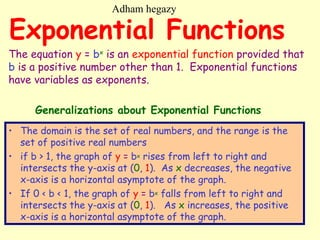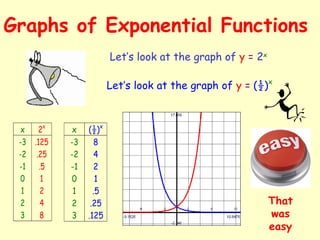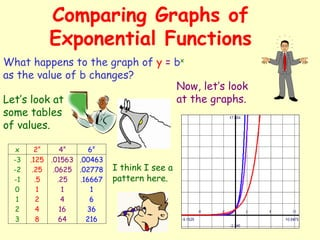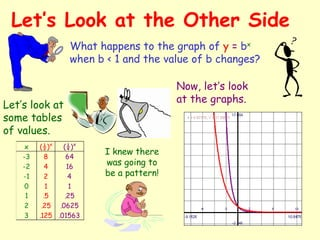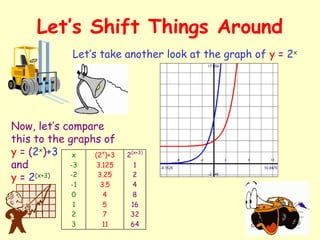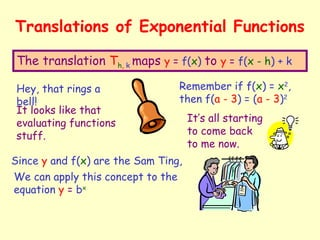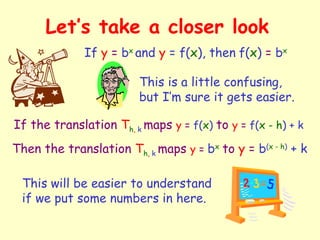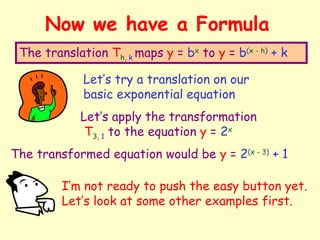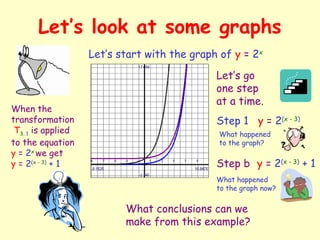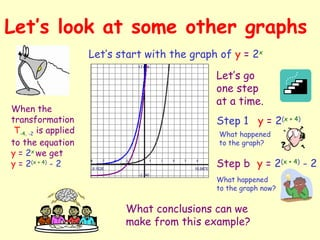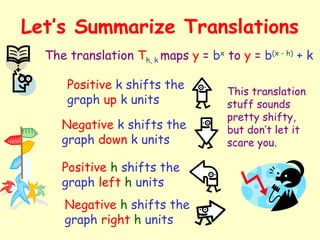1 sur 12
Publicité

### _12_ - Exponential Functions (1).pdf

1. Exponential Functions • The domain is the set of real numbers, and the range is the set of positive real numbers • if b > 1, the graph of y = bx rises from left to right and intersects the y-axis at (0, 1). As x decreases, the negative x-axis is a horizontal asymptote of the graph. • If 0 < b < 1, the graph of y = bx falls from left to right and intersects the y-axis at (0, 1). As x increases, the positive x-axis is a horizontal asymptote of the graph. The equation y = bx is an exponential function provided that b is a positive number other than 1. Exponential functions have variables as exponents. Generalizations about Exponential Functions Adham hegazy
2. Graphs of Exponential Functions Let’s look at the graph of y = 2x x 2x -3 -2 -1 0 1 2 3 .125 .25 .5 1 2 4 8 Let’s look at the graph of y = (½)x x (½)x -3 -2 -1 0 1 2 3 8 4 2 1 .5 .25 .125 That was easy
3. Comparing Graphs of Exponential Functions What happens to the graph of y = bx as the value of b changes? x 2x 4x 6x -3 -2 -1 0 1 2 3 .125 .25 .5 1 2 4 8 .01563 .0625 .25 1 4 16 64 .00463 .02778 .16667 1 6 36 216 Let’s look at some tables of values. Now, let’s look at the graphs. I think I see a pattern here.
4. Let’s Look at the Other Side What happens to the graph of y = bx when b < 1 and the value of b changes? x (½)x (¼)x -3 -2 -1 0 1 2 3 8 4 2 1 .5 .25 .125 64 16 4 1 .25 .0625 .01563 Let’s look at some tables of values. Now, let’s look at the graphs. I knew there was going to be a pattern!
5. Let’s Shift Things Around Let’s take another look at the graph of y = 2x Now, let’s compare this to the graphs of y = (2x )+3 and y = 2(x+3) x -3 -2 -1 0 1 2 3 (2x )+3 3.125 3.25 3.5 4 5 7 11 2(x+3) 1 2 4 8 16 32 64
6. Translations of Exponential Functions The translation Th, k maps y = f(x) to y = f(x - h) + k Hey, that rings a bell! Since y and f(x) are the Sam Ting, It looks like that evaluating functions stuff. Remember if f(x) = x2 , then f(a - 3) = (a - 3)2 It’s all starting to come back to me now. We can apply this concept to the equation y = bx
7. Let’s take a closer look If the translation Th, k maps y = f(x) to y = f(x - h) + k If y = bx and y = f(x), then f(x) = bx This is a little confusing, but I’m sure it gets easier. Then the translation Th, k maps y = bx to y = b(x - h) + k This will be easier to understand if we put some numbers in here.
8. Now we have a Formula The translation Th, k maps y = bx to y = b(x - h) + k Let’s try a translation on our basic exponential equation Let’s apply the transformation T3, 1 to the equation y = 2x The transformed equation would be y = 2(x - 3) + 1 I’m not ready to push the easy button yet. Let’s look at some other examples first.
9. Let’s look at some graphs Let’s start with the graph of y = 2x Let’s go one step at a time. When the transformation T3, 1 is applied to the equation y = 2x we get y = 2(x - 3) + 1 Step 1 y = 2(x - 3) Step b y = 2(x - 3) + 1 What happened to the graph? What happened to the graph now? What conclusions can we make from this example?
10. Let’s look at some other graphs Let’s start with the graph of y = 2x Let’s go one step at a time. When the transformation T-4, -2 is applied to the equation y = 2x we get y = 2(x + 4) - 2 Step 1 y = 2(x + 4) Step b y = 2(x + 4) - 2 What happened to the graph? What happened to the graph now? What conclusions can we make from this example?
11. Let’s Summarize Translations The translation Th, k maps y = bx to y = b(x - h) + k Positive k shifts the graph up k units Negative k shifts the graph down k units Positive h shifts the graph left h units Negative h shifts the graph right h units This translation stuff sounds pretty shifty, but don’t let it scare you.
12. This exponential equation stuff is pretty easy. I feel like jumping for joy! Oh my! I think I’ll just push the easy button. That was easy
Publicité